Courses

# Second Law Of Thermodynamics MCQ Level - 1 (Part - 1)

## 10 Questions MCQ Test Topic wise Tests for IIT JAM Physics | Second Law Of Thermodynamics MCQ Level - 1 (Part - 1)

Description
This mock test of Second Law Of Thermodynamics MCQ Level - 1 (Part - 1) for IIT JAM helps you for every IIT JAM entrance exam. This contains 10 Multiple Choice Questions for IIT JAM Second Law Of Thermodynamics MCQ Level - 1 (Part - 1) (mcq) to study with solutions a complete question bank. The solved questions answers in this Second Law Of Thermodynamics MCQ Level - 1 (Part - 1) quiz give you a good mix of easy questions and tough questions. IIT JAM students definitely take this Second Law Of Thermodynamics MCQ Level - 1 (Part - 1) exercise for a better result in the exam. You can find other Second Law Of Thermodynamics MCQ Level - 1 (Part - 1) extra questions, long questions & short questions for IIT JAM on EduRev as well by searching above.
QUESTION: 1

### A sample of an ideal gas with initial pressure p and volume V is taken through an isothermal expansion proceed during which the change in entropy is found to be ΔS. The universal gas constant is R. Then, the work done by the gas is given by :

Solution:

By ideal gas equation,
pV = nRT     ...(i)
and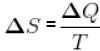...(ii)
Multiplying Equation (i) and (ii),
pVΔQ = nRΔS
⇒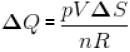∴ For isothermal expansion ΔV = 0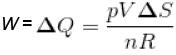QUESTION: 2

### A Carnot engine operating between 27°C and 127°C has efficiency equal to Select one:

Solution:

T1 = (27 + 273)K = 300K
T2 = (127 + 273)K = 400K
Efficiency,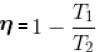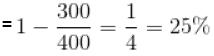QUESTION: 3

### T-S diagram for a Carnot’s cycle is Select one:

Solution:

A Carnot cycle can be represented in a T-S diagram, with T along the y-axis and S (entropy) along the x-axis. The isotherms are parallel horizontal lines at Tc and Th. The isentropes are parallel vertical lines (by definition they are of constant entropy). Thus, a Carnot cycle is represented by a rectangle.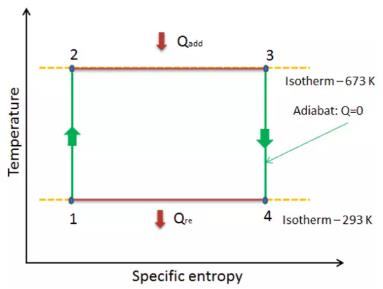QUESTION: 4

The entropy of an isolated system
Select one:

Solution:

The entropy of an isolated system increases in an irreversible process

The second law of thermodynamics states that the entropy of an isolated system never decreases because isolated systems always evolve toward thermodynamic equilibrium, a state with maximum entropy.

Hence the entropy of an isolated system increases in an irreversible process.

QUESTION: 5

A Carnot cycle operates on a working substance between two reservoirs at temperatures T1 and T2 ,  where, T1 > T2 . Amount of heat Q1 is extracted from the reservoir at T1 and an amount Q2 is delivered to the reservoir at T2. Which of the following statements is incorrect?

Solution:

Carnot cycle is a reversible process ideally and in reversible processes the entropy of universe remains constant instead of increasing as in an irreversible process.

QUESTION: 6

Two ends of a rod are kept at 127°C and 227°C. When 2000 cal of heat flows in this rod, then the change in entropy is
Select one:

Solution:

Solution :- T1 = 127 + 273 = 400K

T2 = 227 + 273 = 500K

dT = T2 - T1

dT = 500 - 400 = 100

dS = dQ/dT

dS = 2000/100

dS = 20cal/K

QUESTION: 7

At 0 K, fluids are assumed to have
Select one:

Solution:

Entropy is the measurement of Randomness of a System. Increase in thermal energy increases the randomness and hence Entropy. Now decreasing the temperature of a fluid results in decrease in it's thermal energy which decreases the randomness of the system which in turn decreases the entropy of the fluid. Now there is a finite randomness at normal temperature in the fluid and as we proceed from a higher temperature to a lower temperature the randomness decreases so does the entropy. After continuing this process when we reach 0K further no thermal energy can be extracted from the fluid and hence the system can be said to be completely ordered and as the system is ordered (i.e zero randomness) the entropy is assumed to be zero.

QUESTION: 8

The area of the Carnot cycle on a T-S diagram represents
Select one:

Solution:
QUESTION: 9

A reversible heat engine can have 100% efficiency if the temperature of sink is
Select one:

Solution:
QUESTION: 10

Which of the following is a property of entropy?
Select one:

Solution: